Portfolio Backtesting

2019-06-18

This vignette illustrates the usage of the package portfolioBacktest for automated portfolio backtesting over multiple datasets on a rolling-window basis. It can be used by a researcher/practitioner to backtest a set of different portfolios, as well as a course instructor to assess the students in their portfolio design in a fully automated and convenient manner. The results can be nicely formatted in tables and plots.

Package Snapshot

This package backtests a list of portfolios over multiple datasets on a rolling-window basis, producing final results as in the following.

• Performance table:

• Barplot: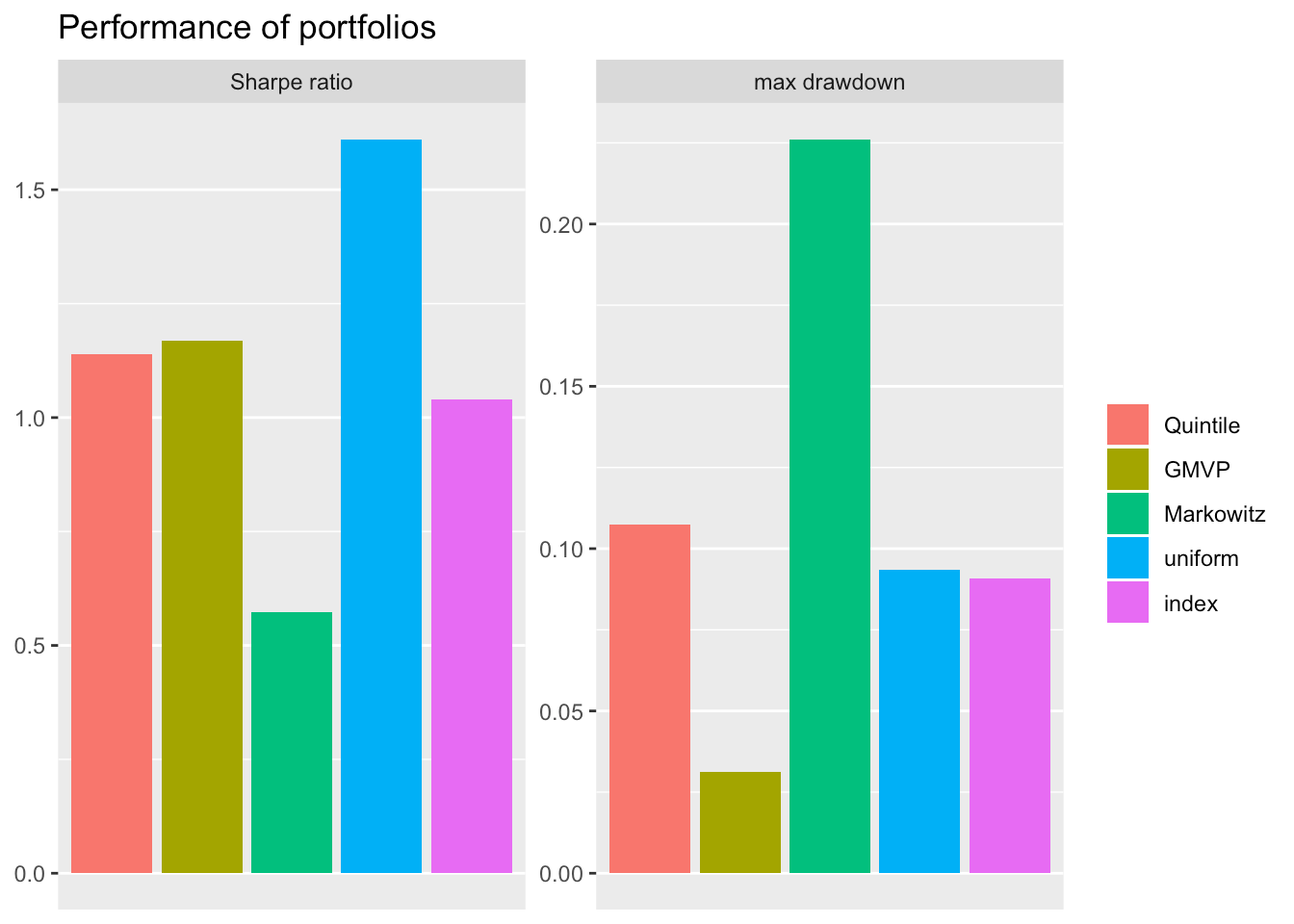• Boxplot: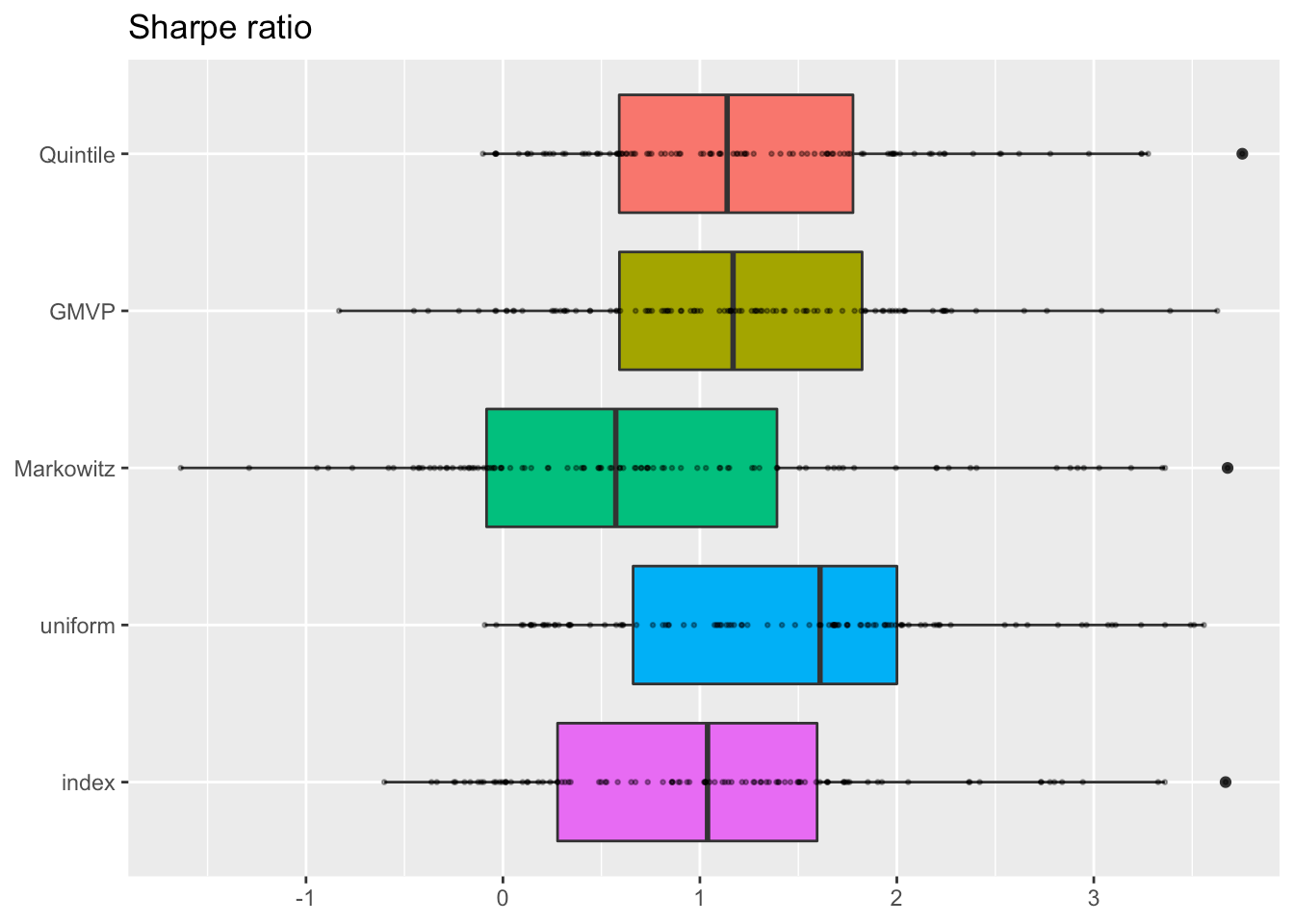Quick Start

Do the backtest on your own portfolio following few steps:

• Step 1 - load package & dataset
library(portfolioBacktest)
data("dataset10")
• Step 2 - define your own portfolio
my_portfolio <- function(dataset) {
prices <- dataset$adjusted N <- ncol(prices) return(rep(1/N, N)) } • Step 3 - do backtest bt <- portfolioBacktest(my_portfolio, dataset10) • Step 4 - check your portfolio performance backtestSummary(bt)$performance
#>                          fun1
#> Sharpe ratio        1.5414027
#> max drawdown        0.0893789
#> annual return       0.1641528
#> annual volatility   0.1218623
#> Sterling ratio      2.2138192
#> Omega ratio         1.2950904
#> ROT bps           696.9894933

Installation

The package can be installed from CRAN or GitHub:

# install stable version from CRAN
install.packages("portfolioBacktest")

# install development version from GitHub
devtools::install_github("dppalomar/portfolioBacktest")

# Getting help
library(portfolioBacktest)
help(package = "portfolioBacktest")
?portfolioBacktest

Basic structure of datasets

The main function portfolioBacktest() requires the argument dataset_list to follow a certain format: it should be a list of several individual datasets, each of them being a list of several xts objects following exactly the same date index. One of those xts objects must contain the historical prices of the stocks, but we can have additional xts objects containing other information such as volume of the stocks or index prices. The package contains a small dataset sample for illustration purposes:

data("dataset10")  # load the embedded dataset
class(dataset10)  # show dataset class
#>  "list"
names(dataset10[1:3])  # show names of a few datasets
#>  "dataset 1" "dataset 2" "dataset 3"
names(dataset10$dataset 1) # structure of one dataset #>  "adjusted" "index" head(dataset10$dataset 1$adjusted[, 1:3]) #> MAS.Adjusted MGM.Adjusted CMI.Adjusted #> 2015-04-24 22.05079 21.34297 121.8492 #> 2015-04-27 22.13499 21.26537 124.3041 #> 2015-04-28 22.68226 21.69223 122.5187 #> 2015-04-29 22.54755 20.47956 123.2150 #> 2015-04-30 22.30337 20.51836 123.4203 #> 2015-05-01 22.85065 20.76090 125.9823 Note that each dataset contains an xts object called "adjusted" (adjusted prices). By default, portfolioBacktest() will use such adjusted prices to calculate the portfolio return. But one can change this setting with the argument price_name in function portfolioBacktest(). Obtaining more data We emphasize that 10 datasets are not enough for properly backtesting portfolios. In this package, we provide the function stockDataDownload() to download online data resources in the required data format. Then, the function stockDataResample() can help resample the downloaded data into multiple datasets (each resample is obtained by randomly choosing a subset of the stock names and randomly choosing a time period over the available long period), which can be directly passed to portfolioBacktest(). We recommend using these two functions to generate multiple datasets for serious backtesting: data("SP500_symbols") # load the SP500 symbols # download data from internet SP500 <- stockDataDownload(stock_symbols = SP500_symbols, from = "2008-12-01", to = "2018-12-01") # resample 10 times from SP500, each with 50 stocks and 2-year consecutive data my_dataset_list <- stockDataResample(SP500_YAHOO, N_sample = 50, T_sample = 252*2, num_datasets = 10) Each individual dataset will contain 7 xts objects with names: open, high, low, close, volume, adjusted, index. Once the datasets are ready they can be saved into a file to avoid having to download and resample every time. Expanding the datasets Additional data can be helpful in designing portfolios. One can add as many other xts objects in each dataset as desired. For example, if the Moving Average Convergence Divergence (MACD) information is needed by the portfolio functions, one can manually add it to the dataset as follows: for (i in 1:length(dataset10)) dataset10[[i]]$MACD <- apply(dataset10[[i]]$adjusted, 2, function(x) { TTR::MACD(x)[ , "macd"] }) Defining Portfolios The portfolios have to be defined in the form of function that takes as input a dataset (which will be automatically windowed during the backtesting following a rolling-window basis) containing a list of xts objects (following the format of the elements of the argument dataset_list) and returns the portfolio as a numerical vector of normalized weights of the same length as the number of stocks. We give the examples for the quintile portfolio, the global minimum variance portfolio (GMVP), and the Markowitz mean-variance portfolio as follows (under practical constraints $$\mathbf{w} \ge \mathbf{0}$$ and $$\mathbf{1}^{T} \mathbf{w} =1$$): # define quintile portfolio quintile_portfolio_fun <- function(data) { X <- diff(log(data$adjusted))[-1]  # compute log returns
N <- ncol(X)
# design quintile portfolio
ranking <- sort(colMeans(X), decreasing = TRUE, index.return = TRUE)$ix w <- rep(0, N) w[ranking[1:round(N/5)]] <- 1/round(N/5) return(w) } # define GMVP (allowing shorting) GMVP_portfolio_fun <- function(data) { X <- diff(log(data$adjusted))[-1]  # compute log returns
Sigma <- cov(X)  # compute SCM
# design GMVP
w <- solve(Sigma, rep(1, nrow(Sigma)))
w <- w/sum(abs(w))
return(w)
}

# define Markowitz mean-variance portfolio
library(CVXR)
Markowitz_portfolio_fun <- function(data) {
X <- diff(log(data$adjusted))[-1] # compute log returns mu <- colMeans(X) # compute mean vector Sigma <- cov(X) # compute the SCM # design mean-variance portfolio w <- Variable(nrow(Sigma)) prob <- Problem(Maximize(t(mu) %*% w - 0.5*quad_form(w, Sigma)), constraints = list(w >= 0, sum(w) == 1)) result <- solve(prob) return(as.vector(result$getValue(w)))
}

Backtesting and Plotting

With the datasets and portfolios ready, we can now do the backtest easily. For example, to obtain the three portfolios’ performance over the datasets, we just need combine them in a list and run the backtest in one line:

portfolios <- list("Quintile"  = quintile_portfolio_fun,
"GMVP"      = GMVP_portfolio_fun,
"Markowitz" = Markowitz_portfolio_fun)
bt <- portfolioBacktest(portfolios, dataset10, benchmark = c("uniform", "index"))

Result format

Here bt is a list storing all the backtest results according to the passed functions list (plus the two benchmarks):

names(bt)
#>  "Quintile"  "GMVP"      "Markowitz" "uniform"   "index"

Each element of bt is also a list storing more information for each of the datasets:

#>                           levelName
#> 1  bt
#> 2   ¦--Quintile
#> 3   ¦   ¦--dataset 1
#> 4   ¦   ¦   ¦--performance
#> 5   ¦   ¦   ¦--cpu_time
#> 6   ¦   ¦   ¦--error
#> 7   ¦   ¦   ¦--error_message
#> 8   ¦   ¦   ¦--portfolio
#> 9   ¦   ¦   ¦--return
#> 10  ¦   ¦   °--cumPnL
#> 11  ¦   ¦--dataset 2
#> 12  ¦   ¦   ¦--performance
#> 13  ¦   ¦   ¦--cpu_time
#> 14  ¦   ¦   ¦--error
#> 15  ¦   ¦   ¦--error_message
#> 16  ¦   ¦   ¦--portfolio
#> 17  ¦   ¦   ¦--return
#> 18  ¦   ¦   °--cumPnL
#> 19  ¦   ¦--dataset 3
#> 20  ¦   ¦   °--... 7 nodes w/ 0 sub
#> 21  ¦   °--... 7 nodes w/ 56 sub
#> 22  °--... 4 nodes w/ 383 sub

One can extract any desired backtest information directly from the returned variable bt.

The package also contains several convenient functions to extract information from the backtest results.

• Select several performance measures of one specific portfolio:
# select sharpe ratio and max drawdown performance of uniform portfolio
backtestSelector(bt, portfolio_name = "Quintile",
measures = c("Sharpe ratio", "max drawdown"))
#> $performance #> Sharpe ratio max drawdown #> dataset 1 1.066989270 0.09384104 #> dataset 2 1.254843826 0.10406013 #> dataset 3 2.582276098 0.06952085 #> dataset 4 1.526569864 0.09921398 #> dataset 5 -0.006082829 0.17328255 #> dataset 6 0.981613296 0.10320105 #> dataset 7 1.778535154 0.08836202 #> dataset 8 -0.242004981 0.27460141 #> dataset 9 1.756090437 0.11288730 #> dataset 10 1.421113265 0.09834212 • Tables of several performance measures of the portfolios (classified by performance criteria): # show the portfolios performance in tables backtestTable(bt, measures = c("Sharpe ratio", "max drawdown")) #>$Sharpe ratio
#>                Quintile        GMVP   Markowitz    uniform       index
#> dataset 1   1.066989270  0.44347512 -0.09535393 1.46290397  1.31332671
#> dataset 2   1.254843826  0.46887119  1.02098962 0.36602873  0.07019635
#> dataset 3   2.582276098  1.46794068  1.16826052 2.47349102  1.84104358
#> dataset 4   1.526569864 -0.04523114  0.20480420 1.23875303  0.86839335
#> dataset 5  -0.006082829  0.73346873 -0.29993326 0.24833981  0.05727968
#> dataset 6   0.981613296  2.13392160  0.55860989 1.87215178  2.63675781
#> dataset 7   1.778535154  4.27893672 -0.36846679 2.61728179  1.60490706
#> dataset 8  -0.242004981  1.12369708  1.00012843 0.08820003 -0.16297323
#> dataset 9   1.756090437  0.82347161  0.64727039 1.61990151  1.35084113
#> dataset 10  1.421113265  1.89578620  0.37927803 2.02390131  1.75889262
#>
#> $max drawdown #> Quintile GMVP Markowitz uniform index #> dataset 1 0.09384104 0.02571868 0.20824525 0.06678369 0.05695256 #> dataset 2 0.10406013 0.04362000 0.23314434 0.13218930 0.12628589 #> dataset 3 0.06952085 0.02271049 0.16813510 0.04800769 0.05848025 #> dataset 4 0.09921398 0.06836597 0.15162912 0.10861575 0.10555293 #> dataset 5 0.17328255 0.06131943 0.64819866 0.11546985 0.10555293 #> dataset 6 0.10320105 0.02250230 0.33947368 0.03869864 0.02819678 #> dataset 7 0.08836202 0.01603770 0.27695971 0.05440115 0.07783609 #> dataset 8 0.27460141 0.04080267 0.28835512 0.19664607 0.18524842 #> dataset 9 0.11288730 0.07093588 0.24967326 0.10097014 0.10555293 #> dataset 10 0.09834212 0.03648148 0.09785354 0.07778765 0.05695256 • Summary of performance measures: bt_sum <- backtestSummary(bt) names(res_sum) #>  "performance_summary" "failure_rate" "cpu_time_summary" #>  "error_message" res_sum$performance_summary
#>                      Quintile        GMVP   Markowitz      uniform
#> Sharpe ratio        1.1382727  1.16856836   0.5729870   1.61004827
#> max drawdown        0.1073746  0.03132534   0.2259520   0.09360759
#> annual return       0.1939239  0.04870919   0.1494533   0.19214798
#> annual volatility   0.1613030  0.04179758   0.3165205   0.13532259
#> Sterling ratio      1.8075257  1.43035842   0.8001927   2.19665444
#> Omega ratio         1.2059942  1.21518329   1.1245883   1.29231636
#> ROT bps           253.2452187 81.72844616 187.1631221 837.50477655
#>                        index
#> Sharpe ratio      1.03893289
#> max drawdown      0.09071472
#> annual return     0.13232931
#> annual volatility 0.12487000
#> Sterling ratio    1.37615448
#> Omega ratio       1.20714690
#> ROT bps                  Inf

For more flexible usage of these functions, one can refer to the help pages of these functions.

Besides, the package also provides some functions to show results in tables and figures.

• Performance table:
summaryTable(res_sum, type = "DT", order_col = 2, order_dir = "desc")

• Barplot:
summaryBarPlot(bt_sum, measures = c("Sharpe ratio", "max drawdown"))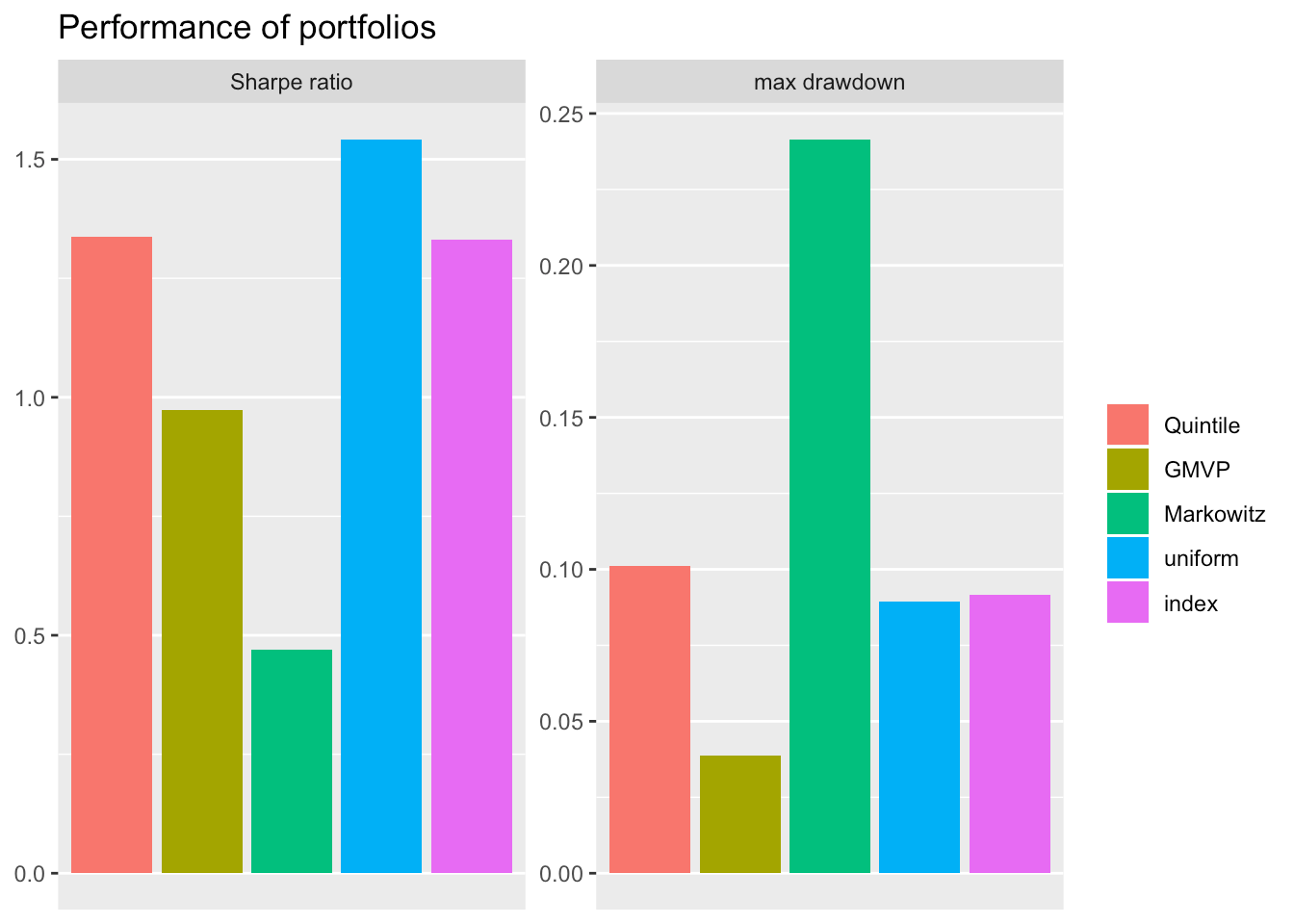• BoxPlot:
backtestBoxPlot(bt, measure = "Sharpe ratio")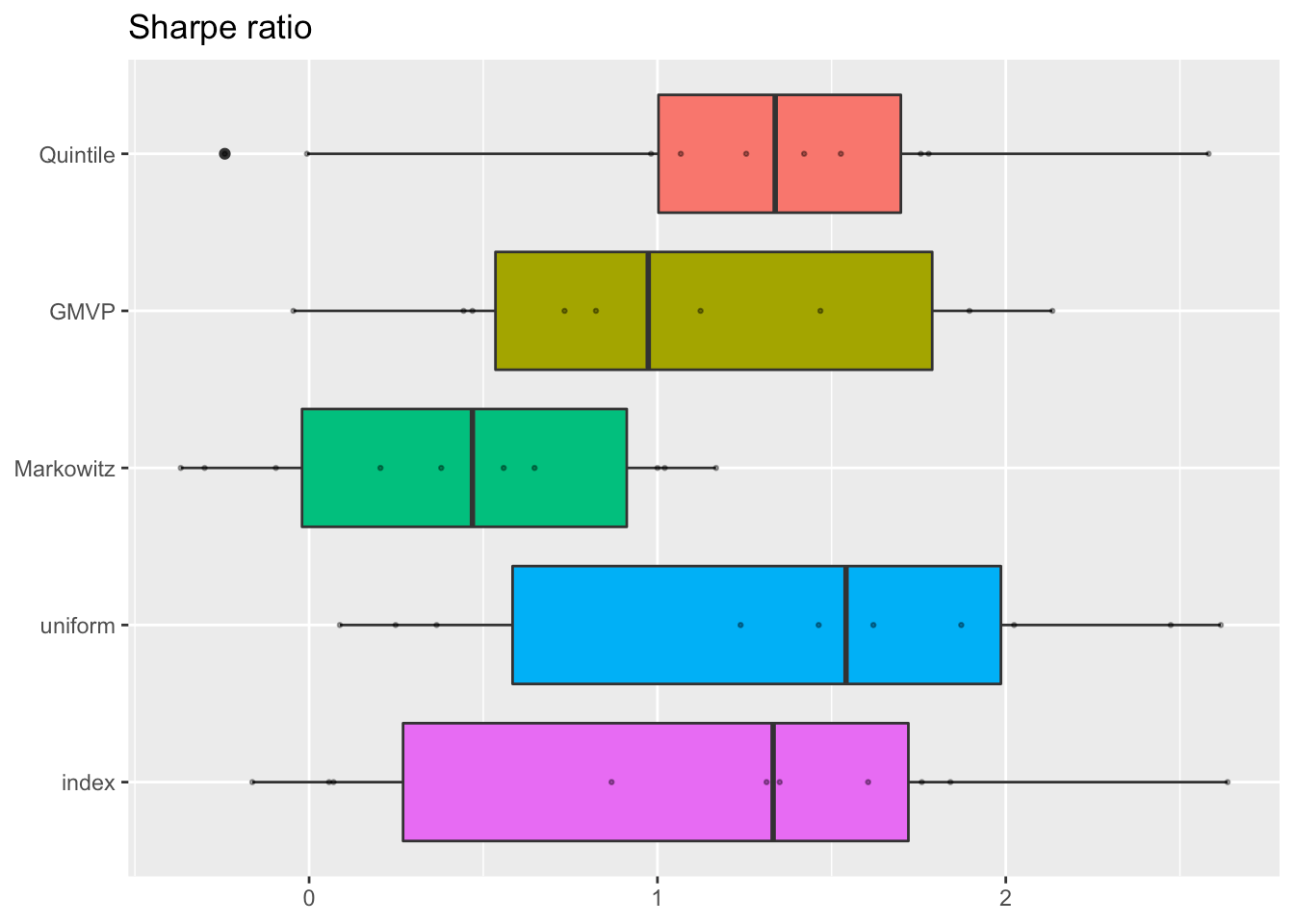Incoporating benchmarks

When performing the backtest of the designed portfolio functions, one may want to incorporate some benchmarks. The package currently suppports two benchmarks: uniform portfolio and index of the market. (Note that to incorporate the index benchmark each dataset needs to contain one xts object named index.) Once can easily choose the benchmarks by passing the corresponding value to argument benchmark:

bt <- portfolioBacktest(portfolios, dataset10, benchmark = c("uniform", "index"))
names(bt)
#>  "Quintile"  "GMVP"      "Markowitz" "uniform"   "index"

Progress bar

In order to monitor the backtest progress, one can choose to turn on a progress bar by setting the argument show_progress_bar:

bt <- portfolioBacktest(portfolios, dataset10, show_progress_bar = TRUE)

Parallel backtesting

The backtesting typically incurs in a very heavy computational load when the number of portfolios or datasets is large (also depending on the computational cost of each portfolio function). The package contains support for parallel computational mode. Users can choose to evaluate different portfolio functions in parallel or, in a more fine-grained way, to evaluate multiple datasets in parallel for each function:

portfun <- Markowitz_portfolio_fun

# parallel = 2 for functions
system.time(
bt_nopar <- portfolioBacktest(list(portfun, portfun), dataset10)
)
#>    user  system elapsed
#>  40.376   0.380  40.840
system.time(
bt_parfuns <- portfolioBacktest(list(portfun, portfun), dataset10,
paral_portfolios = 2)
)
#>    user  system elapsed
#>   0.432   0.148  23.610

# parallel = 5 for datasets
system.time(
bt_nopar <- portfolioBacktest(portfun, dataset10)
)
#>    user  system elapsed
#>  19.178   0.090  19.340
system.time(
bt_pardata <- portfolioBacktest(portfun, dataset10,
paral_datasets = 5)
)
#>    user  system elapsed
#>   1.038   0.310  12.431

It is obvious that the evaluation time for backtesting has been significantly reduced. Note that the parallel evaluation elapsed time will not be exactly equal to the original time divided by parallel cores because starting new R sessions also takes extra time. Besides, the two parallel modes can be simultaneous used.

Tracing where execution errors happen

Execution errors during backtesting may happen unexpectedly when executing the different portfolio functions. Nevertheless, such errors are properly catched and bypassed by the backtesting function portfolioBacktest() so that the execution of the overall backtesting is not stopped. For debugging purposes, to help the user trace where and when the execution errors happen, the result of the backtesting contains all the necessary information about the errors, including the call stack when a execution error happens. Such information is given as the attribute error_stack of the returned error_message.

For example, let’s define a portfolio function that will throw a error:

sub_function2 <- function(x) {
"a" + x  # an error will happen here
}

sub_function1 <- function(x) {
return(sub_function2(x))
}

wrong_portfolio_fun <- function(data) {
N <- ncol(data$adjusted) uni_port <- rep(1/N, N) return(sub_function1(uni_port)) } Now, let’s pass the above portfolio function to portfolioBacktest() and see how to check the error trace: bt <- portfolioBacktest(wrong_portfolio_fun, dataset10) res <- backtestSelector(bt, portfolio_index = 1) # information of 1st error error1 <- res$error_message[]
str(error1)
#>  chr "non-numeric argument to binary operator"
#>  - attr(*, "error_stack")=List of 2
#>   ..$at : chr "\"a\" + x" #> ..$ stack: chr "sub_function1(uni_port)\nsub_function2(x)"

# the exact location of error happening
cat(attr(error1, "error_stack")$at) #> "a" + x # the call stack of error happening cat(attr(error1, "error_stack")$stack)
#> sub_function1(uni_port)
#> sub_function2(x)

Backtesting over files: usage for grading students

In some situations, one may have to backtest portfolios from different sources stored in different files, e.g., students in a porftolio design course (in fact, this package was originally developed to assess students in the course “Portfolio Optimization with R” from the MSc in Financial Mathematics (MAFM)). In such cases, the different portfolios may have conflicting dependencies and loading all of them into the environment may not be a reasonable approach. The package adds support for backtesting portfolios given in individual files in a folder in a way that each is executed in a clean environment without affecting each other. It suffices to write each portfolio function into an R script (with unique filename) containing the portfolio function named exactly portfolio_fun() as well as any other auxiliary functions that it may require (needless to say that the required packages should be loaded in that script with library()). All theses files should be put into a file folder, whose path will be passed to the function portfolioBacktest() with the argument folder_path.

If an instructor wants to evaluate students of a course in their portfolio design, this can be easily done by asking each student to submit an R script with a unique filename like STUDENTNUMBER.R. For example, suppose we have three files in the folder portfolio_files named 0001.R, 0002.R, and 0003.R. Then:

bt_all_students <- portfolioBacktest(folder_path = "portfolio_files",
dataset = dataset10)
names(bt_all_students)
#>  "0001" "0002" "0003"

Leaderboard of portfolios with user-defined ranking

Now we can rank the different portfolios/students based on a weighted combination of the rank percentiles (termed scores) of the performance measures:

leaderboard <- backtestLeaderboard(bt_all_students,
weights = list("Sharpe ratio"  = 7,
"max drawdown"  = 1,
"annual return" = 1,
"ROT bps"       = 1))

library(gridExtra)
grid.table(leaderboard$leaderboard_scores)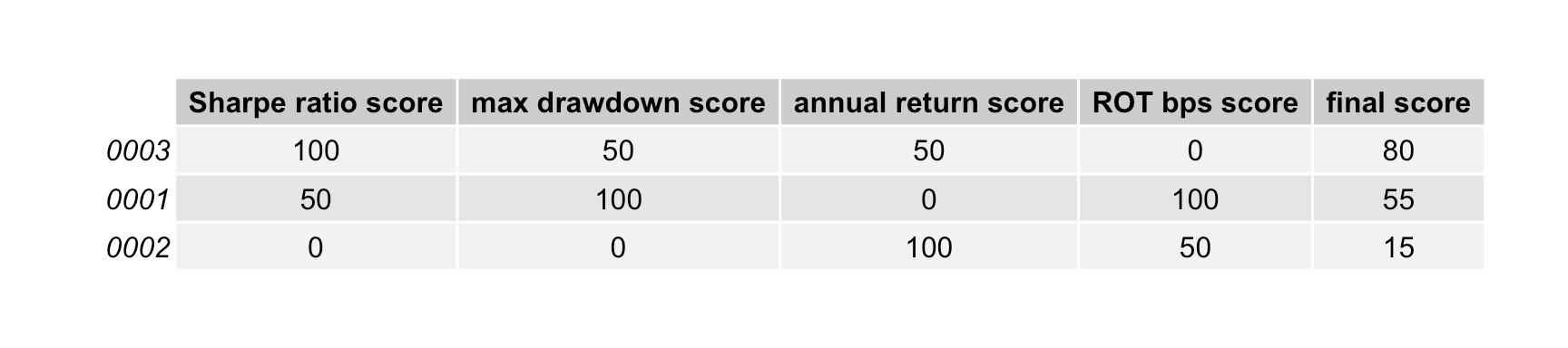Example of a script file to be submitted by a student Consider the student with id number 666. Then the script file should be named 666.R and should contain the portfolio function called exactly portfolio_fun() as well as any other auxiliary functions that it may require (and any required package loading with library()): library(CVXR) auxiliary_function <- function(x) { # here whatever code } portfolio_fun <- function(prices) { X <- as.matrix(diff(log(data$adjusted))[-1])  # compute log returns
mu <- colMeans(X)  # compute mean vector
Sigma <- cov(X)  # compute the SCM
# design mean-variance portfolio
w <- Variable(nrow(Sigma))
prob <- Problem(Maximize(t(mu) %*% w - 0.5*quad_form(w, Sigma)),
constraints = list(w >= 0, sum(w) == 1))
result <- solve(prob)
return(as.vector(result\$getValue(w)))
}

Appendix

Performance criteria

The performance criteria currently considered by the package are:

• annual return: the annualized return
• annual volatility: the annualized standard deviation of returns
• max drawdown: the maximum drawdown is defined as the maximum loss from a peak to a trough of a portfolio
• Sharpe ratio: annualized Sharpe ratio, the ratio between annualized return and annualized standard deviation
• Sterling ratio: the return over average drawdown, see here for complete definition. In the package, we use $\text{Sterling ratio} = \frac{\text{annualized return}}{\text{max drawdown}}$
• Omega ratio: the probability weighted ratio of gains over losses for some threshold return target, see here for complete definition. The ratio is calculated as: $\Omega(r) = \frac{\int_{r}^{\infty} (1-F(x))dx}{\int_{-\infty}^{r} F(x)dx}$ In the package, we use $$\Omega(0)$$, which is also known as Gain-Loss-Ratio.
• ROT bps: Return over Turnover (ROT) defined as the sum of cummulative return over the sum of turnover.

Future features

The package currently has the following limitations that we plan to address in the future:

• Transaction cost: the transaction cost is currently not accounted for in the computation of the portfolio returns during the backtesting (although the ROT can currently be conveniently used to assess the potential effects of a high turnover in terms of transaction costs).
• Turnover term: the portfolio function currently only receives as argument the dataset, but not the currently held portfolio $$\mathbf{w}_{t-1}$$, so the the turnover $$\lVert \mathbf{w}_{t} - \mathbf{w}_{t-1} \rVert _{1}$$ cannot be taken into account in the design.
• Additional performance measures: there are countless of additional performance measures that could be included such as the Sortino ratio.